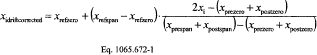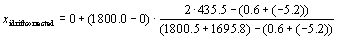# Title 40

## SECTION 1065.672

### 1065.672 Drift correction.

§ 1065.672 Drift correction.

(a) Scope and frequency. Perform the calculations in this section to determine if gas analyzer drift invalidates the results of a test interval. If drift does not invalidate the results of a test interval, correct that test interval's gas analyzer responses for drift according to this section. Use the drift-corrected gas analyzer responses in all subsequent emission calculations. Note that the acceptable threshold for gas analyzer drift over a test interval is specified in § 1065.550 for both laboratory testing and field testing.

(b) Correction principles. The calculations in this section utilize a gas analyzer's responses to reference zero and span concentrations of analytical gases, as determined sometime before and after a test interval. The calculations correct the gas analyzer's responses that were recorded during a test interval. The correction is based on an analyzer's mean responses to reference zero and span gases, and it is based on the reference concentrations of the zero and span gases themselves. Validate and correct for drift as follows:

(c) Drift validation. After applying all the other corrections - except drift correction - to all the gas analyzer signals, calculate brake-specific emissions according to § 1065.650. Then correct all gas analyzer signals for drift according to this section. Recalculate brake-specific emissions using all of the drift-corrected gas analyzer signals. Validate and report the brake-specific emission results before and after drift correction according to § 1065.550.

(d) Drift correction. Correct all gas analyzer signals as follows:

(1) Correct each recorded concentration, xi, for continuous sampling or for batch sampling, x .

(2) Correct for drift using the following equation:Where: xidriftcorrected = concentration corrected for drift. xrefzero = reference concentration of the zero gas, which is usually zero unless known to be otherwise. xrefspan = reference concentration of the span gas. xprespan = pre-test interval gas analyzer response to the span gas concentration. xpostspan = post-test interval gas analyzer response to the span gas concentration. xi or x = concentration recorded during test, before drift correction. xprezero = pre-test interval gas analyzer response to the zero gas concentration. xpostzero = post-test interval gas analyzer response to the zero gas concentration. Example: xrefzero = 0 µmol/mol xrefspan = 1800.0 µmol/mol xprespan = 1800.5 µmol/mol xpostspan = 1695.8 µmol/mol xi or x = 435.5 µmol/mol xprezero = 0.6 µmol/mol xpostzero = −5.2 µmol/molxidriftcorrected = 450.2 µmol/mol

(3) For any pre-test interval concentrations, use concentrations determined most recently before the test interval. For some test intervals, the most recent pre-zero or pre-span might have occurred before one or more previous test intervals.

(4) For any post-test interval concentrations, use concentrations determined most recently after the test interval. For some test intervals, the most recent post-zero or post-span might have occurred after one or more subsequent test intervals.

(5) If you do not record any pre-test interval analyzer response to the span gas concentration, xprespan, set xprespan equal to the reference concentration of the span gas:

xprespan = xrefspan.

(6) If you do not record any pre-test interval analyzer response to the zero gas concentration, xprezero, set xprezero equal to the reference concentration of the zero gas:

xprezero = xrefzero.

(7) Usually the reference concentration of the zero gas, xrefzero, is zero: xrefzero = 0 µmol/mol. However, in some cases you might know that xrefzero has a non-zero concentration. For example, if you zero a CO2 analyzer using ambient air, you may use the default ambient air concentration of CO2, which is 375 µmol/mol. In this case, xrefzero = 375 µmol/mol. Note that when you zero an analyzer using a non-zero xrefzero, you must set the analyzer to output the actual xrefzero concentration. For example, if xrefzero = 375 µmol/mol, set the analyzer to output a value of 375 µmol/mol when the zero gas is flowing to the analyzer.

[70 FR 40516, July 13, 2005, as amended at 74 FR 8427, Feb. 24, 2009; 75 FR 23056, Apr. 30, 2010]